Three wires A,B,C made of the same material and radius have different lengths. The graphs in the figure show the elongation-load variation. The longest wire is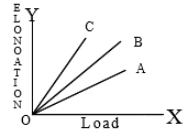1. A

2. B

3. C

4. All

Concept Questions :-

Stress-strain curve
High Yielding Test Series + Question Bank - NEET 2020

Difficulty Level:

The strain-stress curves of three wires of different materials are shown in the figure. P, Q and R are the elastic limits of the wires. The figure shows that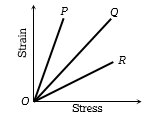(a) Elasticity of wire P is maximum

(b) Elasticity of wire Q is maximum

(c) Tensile strength of R is maximum

(d) None of the above is true

Concept Questions :-

Stress-strain curve
High Yielding Test Series + Question Bank - NEET 2020

Difficulty Level:

The diagram shows a force-extension graph for a rubber band. Consider the following statements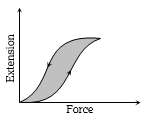I. It will be easier to compress this rubber than expand it

II. Rubber does not return to its original length after it is stretched

III. The rubber band will get heated if it is stretched and released

Which of these can be deduced from the graph?

(a)   III only                               (b)   II and III

(c)   I and III                             (d)   I only

Concept Questions :-

Stress-strain curve
High Yielding Test Series + Question Bank - NEET 2020

Difficulty Level:

The adjacent graph shows the extension $\left(∆l\right)$ of a wire of length 1m suspended from the top of a roof at one end with a load W connected to the other end. If the cross sectional area of the wire is ${10}^{-6}{m}^{2}$ calculate the young’s modulus of the material of the wire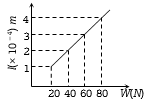(a) $2×{10}^{11}N/{m}^{2}$

(b) $2×{10}^{-11}N/{m}^{2}$

(c) $3×{10}^{-12}N/{m}^{2}$

(d) $2×{10}^{-13}N/{m}^{2}$

Concept Questions :-

Stress-strain curve
High Yielding Test Series + Question Bank - NEET 2020

Difficulty Level:

The graph shows the behaviour of a length of wire in the region for which the substance obeys Hook’s law. P and Q represent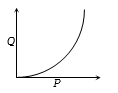(a) P = applied force, Q = extension

(b) P = extension, Q = applied force

(c) P = extension, Q = stored elastic energy

(d) P = stored elastic energy, Q = extension

Concept Questions :-

Stress-strain curve
High Yielding Test Series + Question Bank - NEET 2020

Difficulty Level:

The diagram shows stress v/s strain curve for the materials A and B. From the curves we infer that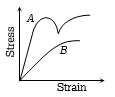(a) A is brittle but B is ductile

(b) A is ductile and B is brittle

(c) Both A and B are ductile

(d) Both A and B are brittle

Concept Questions :-

Stress-strain curve
High Yielding Test Series + Question Bank - NEET 2020

Difficulty Level:

The stress versus strain graphs for wires of two materials A and B are as shown in the figure. If ${Y}_{A}$ and ${Y}_{B}$ are the Young ‘s modulii of the materials, then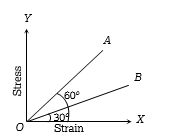(a) ${Y}_{B}=2{Y}_{A}$

(b) ${Y}_{A}={Y}_{B}$

(c) ${Y}_{B}=3{Y}_{A}$

(d) ${Y}_{A}=3{Y}_{B}$

Concept Questions :-

Stress-strain curve